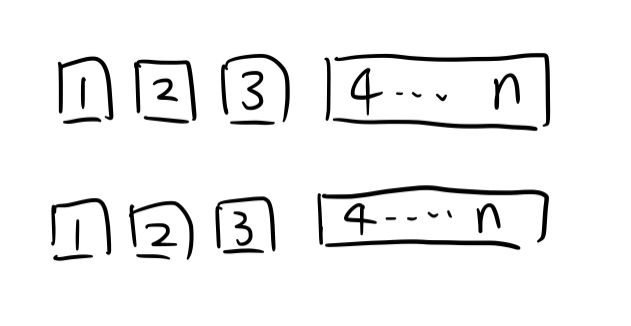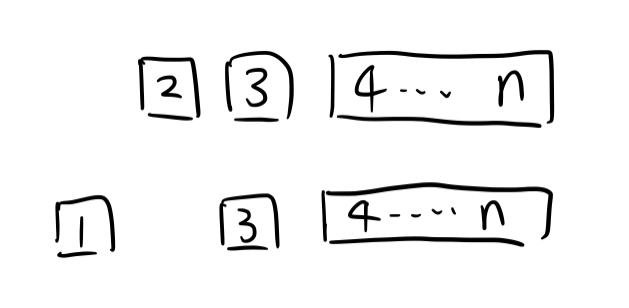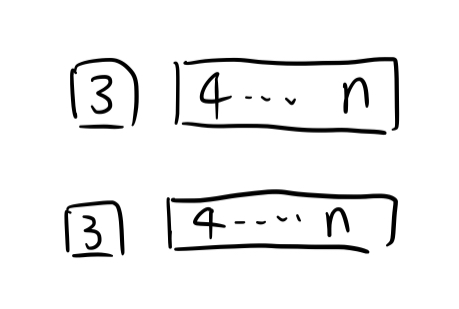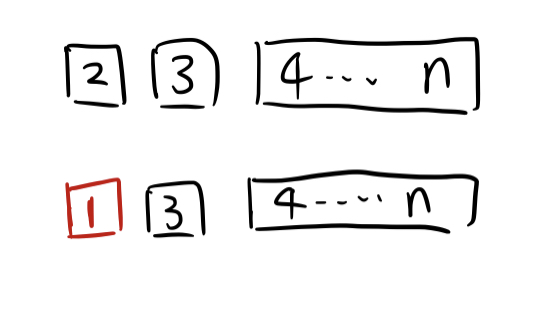## 公式

### 组合数 $C(n,m)$

1. $C_n^0 = C_n^n = 1$
2. $C_n^k = C_{n-1}^k + C_{n-1}^{k-1}$
3. $C_n^k = \frac{n!}{k!(n-k)!}$

$n$ 个中选 $k$ 个， 考虑 $n$ 个元素中的第一个元素：

1. 如果它被选中，有 $C_{n-1}^{k-1}$ 种。
2. 如果它没有被选中，有 $C_{n-1}^k$ 种。

### 二项式定理

$(a+b)^n = \sum\limits_{k=0}^n C_n^ka^kb^{n-k}$

### 卡特兰数 (Catalan)

1. $H_n = 1 ~ (n=0,1)$

2. $H_n = \frac{C_{2n}^n}{n+1}~(n \geq 2)$

3. $H_n = C_{2n}^n - C_{2n}^{n-1}$

1. $H_n = \sum\limits_{i=0}^{n-1}H_{i}H_{n-i-1} = H_0H_{n-1} + H_1H_{n-2} + … + H_{n-1}H_0$

2. $H_n = \frac{(4n-2)}{n+1} H_{n-1}$

### 第二类斯特林数

$S(n,m)$ 代表将 $n$ 个不同的小球，放进 $m$ 个相同，非空盒子的方案数

$S(n,m) = \sum\limits_{i=0}^m (-1)^{m-i}\frac{i^n}{i!(m-i)!}$

$S(n,m) = m*S(n-1,m) + S(n-1,m-1)$

1. 独占一个盒子：相当于，其他 $n-1$ 个小球要放进 $m-1$ 个盒子中，且盒子不为空，所以为 $S(n-1,m-1)$
2. 不独占一个盒子：相当于，先将其他 $n-1$ 个小球放进 $m$ 个盒子中，且盒子不为空，然后从 $m$ 个盒子中选一个，把当前小球放进去，所以为 $m*S(n-1,m)$

## 经典例题

### 例1 男女生排列问题

1. 如果女生必须全排在一起，有多少种排法？

2. 如果女生不能相邻，有多少种排法？

3. 如果两端都不排女生，有多少种排法？

4. 如果两端不都排女生，有多少种排法？

### 例2 小球放盒子问题

$C_{n-1}^{m-1}$

$C_{n+m-1}^{m-1}$

$S(n,m) = m*S(n-1,m) + S(n-1,m-1)$

$\sum\limits_{i=1}^{\min(n,m)}S(n,i)$

$S(n,m) * m!$

$n^m$

### 例3 错排问题

$D_n = (n-1)(D_{n-1} + D_{n-2})$，其中 $D_1 = 0, D_2 = 1$1. 如果 $2 \rightarrow 1$，那么就会变成下图，即 $f(n-2)$ 种。2. 如果 $2 \rightarrow 3 ~ or ~ 4 ~ or ~ … ~ n$，就相当于 $2$ 和 $1$ 必须错开，那就相当于下图，即 $f(n-1)$ 种。